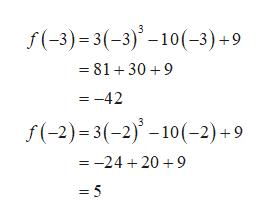# Use the Intermediate Value Theorem to show that the given function: f(x)= 3x3-10x+9 has a zero in the given interval [-3,-2].

Question
6 views

Use the Intermediate Value Theorem to show that the given function:

f(x)= 3x3-10x+9 has a zero in the given interval [-3,-2].

check_circle

Step 1
Step 2help_outlineImage Transcriptionclosef(-3)= 3(-3)° –10(-3)+9 = 81+ 30 +9 = -42 f(-2)= 3(-2)' – 10(-2)+9 = -24 + 20 + 9 = 5 fullscreen

### Want to see the full answer?

See Solution

#### Want to see this answer and more?

Solutions are written by subject experts who are available 24/7. Questions are typically answered within 1 hour.*

See Solution
*Response times may vary by subject and question.
Tagged in

### Algebra